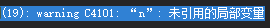# 详解C/C++中const关键字的用法及其与宏常量的比较

更新时间：2017年07月18日 14:27:10   作者：滴巴戈

1.const关键字的性质

2.修饰局部变量

``` const int a = 10;
int const b = 20;```` const chsr* p = "qwerty"; //const用于修饰常量静态字符串`

３．修饰指针

``` const int * n　＝　＆ａ;
int const * n　＝　＆ａ;```

１）常量指针通过不能这个指针改变变量的值，但是可以通过其他的引用来改变变量的值的。

```const int *n = &a;
*n = b;`````` int c = 3;
const int *n = &a;
a = 10;
a = c;```

２）常量指针指向的值不能改变，但是指针本身可以改变，即常量指针可以指向其他的地址。

```　　int a = 1;
int b = 2;
const int *n = &a;
n = &b;```

``` int a = 1;
int b = 2;
int * const n = &a;
*n = b;
b = a;```

```int a = 1;
int b = 2;
int c = 3;
int * const n = &a;
n = &b;```

``` const int * const p= &a;
int const * const p= &a;```

（这种用法在C和C++中是相同的。）

4.修饰引用

```int a = 1;
int const &a = b;
const int &a = b;```

5.函数中使用const

（1）修饰函数参数

` void StrCopy(char *strdes, const char *strsrc);//防止修改指针指向的内容`

` void swap ( int * const p1 , int * const p2 ) //防止修改指针指向的地址`

`void test ( const int * const p1 , const int * const p2 ) //以上两种的结合`

```void function(const Class& Var); //引用参数在函数内不可以改变
void function(const TYPE& Var); //引用参数在函数内为常量不可变```

（这样的一个const引用传递和最普通的函数按值传递的效果是一模一样的,他禁止对引用的对象的一切修改,唯一不同的是按值传递会先建立一个类对象的副本, 然后传递过去,而它直接传递地址,所以这种传递比按值传递更有效.另外只有引用的const传递可以传递一个临时对象,因为临时对象都是const属性, 且是不可见的,他短时间存在一个局部域中,所以不能使用指针,只有引用的const传递能够捕捉到这个家伙。）

（2）修饰函数返回值

```const int * fun2() //调用时 const int *pValue = fun2();
//我们可以把fun2()看作成一个变量，即指针内容不可变。
c.int* const fun3() //调用时 int * const pValue = fun2();
//我们可以把fun2()看作成一个变量，即指针本身不可变。
const int fun1() //这个其实无意义，因为参数返回本身就是赋值。```

6.修饰类相关

（1）用const修饰的类成员变量，只能在类的构造函数初始化列表中赋值，不能在类构造函数体内赋值。

```class A
{
public：
A(int x) : a(x) // 正确
{
//a = x; // 错误
}
private：
const int a;
};```

（2）const修饰成员函数

```class A
{
public:
int& getValue() const
{
// a = 10; // 错误
return a;
}
private:
int a;   // 非const成员变量
};```

a. const成员函数不被允许修改它所在对象的任何一个数据成员。

b. const成员函数能够访问对象的const成员，而其他成员函数不可以。

（3）const修饰类对象/对象指针/对象引用

```class A
{
public:
void funcA() {}
void funcB() const {}
};
int main
{
const A a;
a.funcB(); // 正确
a.funcA(); // X

const A* b = new A();
b->funcB(); // 正确
b->funcA(); // X
}```

（4）在类内重载成员函数

```class A
{
public:
void func() {}
void func() const {} // 重载
};```

```class A
{
public：
A(int size)
: _size(size) // 正确
{}
private：
const int _size;
};
A a(10); //对象a的_size值为10
A b(20); //对象b的_size值为20```

```class A
{
public：
enum{SIZE1 = 10, SIZE2 = 20};//枚举常量
private：
int arr1[SIZE1];
int arr2[SIZE2];
};```

7.修饰全局变量

8.const常量与宏常量的区别

（1）.便于进行类型检查

const常量有数据类型，而宏常量没有数据类型。编译器可以对前者进行类型安全检查，而对后者只进行字符替换，没有类型安全检查，并且在字符替换时可能会产生意料不到的错误（边际效应）。

```//例子：
void f(const int i) { .........} //对传入的参数进行类型检查，不匹配进行提示```

（2）可以节省空间，避免不必要的内存分配

const定义常量从汇编的角度来看，只是给出了对应的内存地址，而不是象#define一样给出的是立即数，所以，const定义的常量在程序运行过程中只有一份拷贝，而#define定义的常量在内存中有若干个拷贝。

```#define PI 3.14159   //常量宏
const doulbe Pi=3.14159; //此时并未将Pi放入ROM中
......
double i=Pi; //此时为Pi分配内存，以后不再分配！
double I=PI; //编译期间进行宏替换，分配内存
double j=Pi; //没有内存分配
double J=PI; //再进行宏替换，又一次分配内存！```

（3）提高了效率

宏定义是一个“编译时”概念，在预处理阶段展开，不能对宏定义进行调试，生命周期结束于编译时期。const常量是一个“运行时”概念，在程序运行时使用，类似于一个只读数据。

（4）可以保护被它修饰的东西

`void f(const int i) { i=10;//error! } //如果在函数体内修改了i，编译器就会报错 `

（5）为函数重载提供了一个参考

``` class A
{
......
void f(int i)  {......} //一个函数
void f(int i) const {......} //上一个函数的重载
......
};```

（6）定义域不同

``` void f1 ()
{
#define N 12
const int n 12;
}
void f2 ()
{
cout<<N <<endl; //正确，N已经定义过，不受定义域限制
cout<<n <<endl; //错误，n定义域只在f1函数中。若想在f2中使用需定义为全局的
}```

（7）做函数参数

宏定义不能作为参数传递给函数；const常量可以在函数的参数列表中出现。

9.const_cast

const_cast运算符用来修改类型的const或volatile属性。

（1）常量指针被转化成非常量的指针，并且仍然指向原来的对象；

（2）常量引用被转换成非常量的引用，并且仍然指向原来的对象。

``` void func()
{
const int a = 10;
int* p = const_cast<int*> (&a);
*p = 20;
std::cout<<*p; // 20
std::cout<<a;  // 10
}```